### Home > INT2 > Chapter 3 > Lesson 3.2.1 > Problem3-74

3-74.

Solve for the missing lengths in the sets of similar figures below.

1. $ΔABC\simΔOPQ$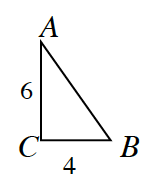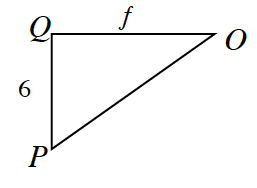$\frac{6}{f} = \frac{4}{6}$

$f=9$

2. $EFGHI\sim STUVW$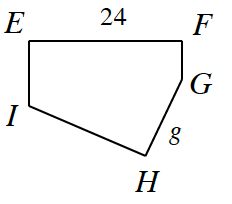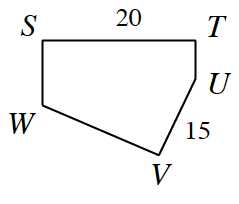$\frac{24}{20} = \frac{g}{15}$

3. $JKLM\sim WXYZ$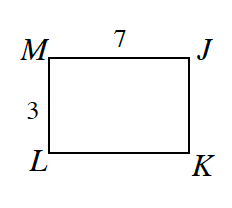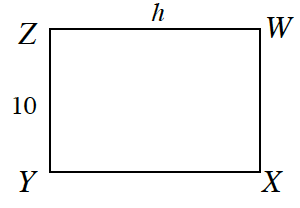Use the same method as parts (a) and (b).

4. Specify a sequence of transformations to map $ΔABC$ onto $ΔOPQ$ in part (a).